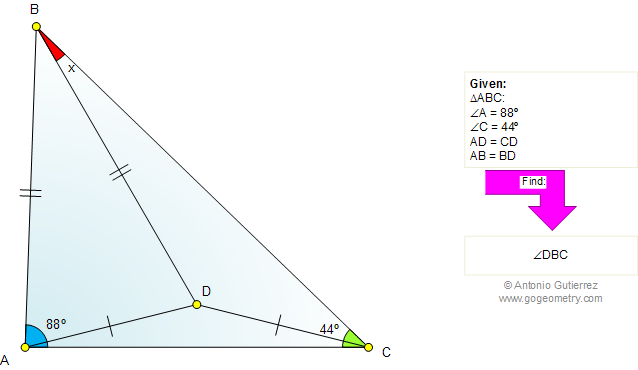# Geometry Problem 727: Triangle, Isosceles, Congruence, Angles, Measurement. Level: High School, Honors Geometry, College, Mathematics Education

 The figure shows a triangle ABC with angle A = 88 degrees and angle C = 44 degrees. AD = CD and AB = BD. Find the measure of the angle DBC.Home | Search | Geometry | Problems | All Problems | Open Problems | Visual Index | 721-730 | Triangles | Congruence | Isosceles Triangle | Angles | Email | Post a comment or solution | By Antonio Gutierrez